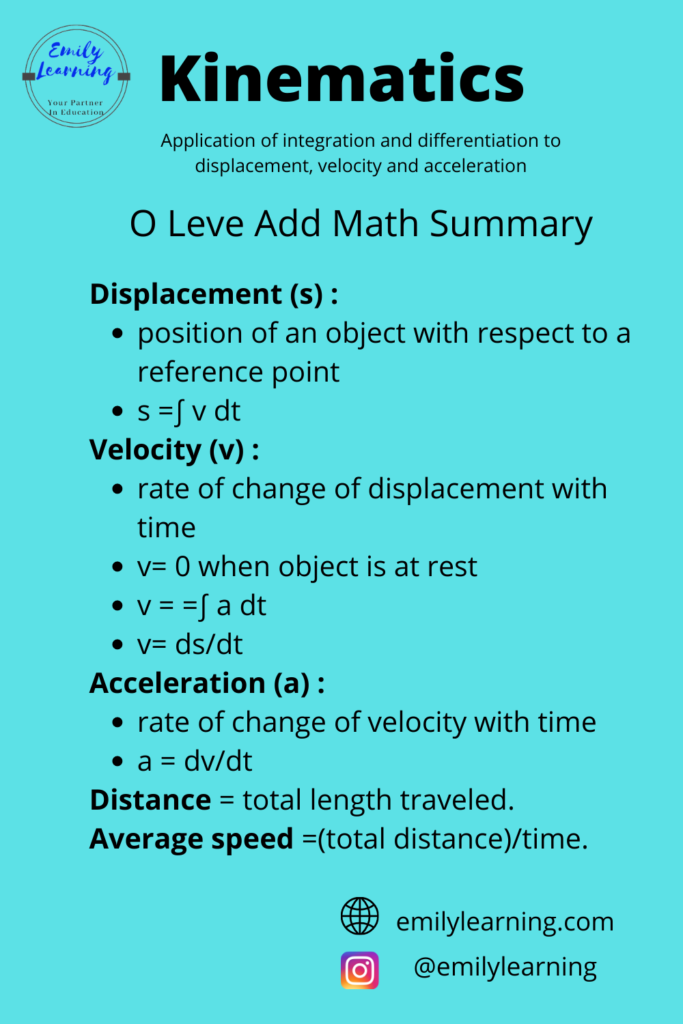# Summary Notes for Kinematics – O Level Add MathHere, you’ll find the summary notes for kinematics written based on the O Level Add Maths syllabus. In my previous articles, I talked in detail here about what you need to know about kinematics for O Levels.

In the chapter on kinematics, you need to know about displacement, velocity, and acceleration in detail, and how to apply differentiation and integration to find the expression of them.

### Summary:

• Displacement (s)
• position of an object with respect to a reference point
• can be found by integrating velocity
• Velocity (v)
• rate of change of displacement with time
• v = 0 hen object is at rest
• can be found by differentiating displacement (s) or integrating acceleration (a)
• Acceleration (a)
• rate of change of velocity with time
• can be found by differentiating velocity

Apart from displacement, velocity, and acceleration, you are also expected to find other physical quantities such as distance and average speed.

• Distance is the total length traveled.
• Average speed is the total distance traveled divided by total time.

You can find the summary of kinematics (application of integration and differentiation to displacement, velocity, and acceleration) in the diagram below:

## Learn O Level Additional Math on-demand

Want to learn O Level Additional Mathematics on-demand? Check out our course here.

error: Content is protected !!